Question

# And its row echelon form (verify ) is given by 1-3 4-2 5 0 01 3- what is the nullity of A without...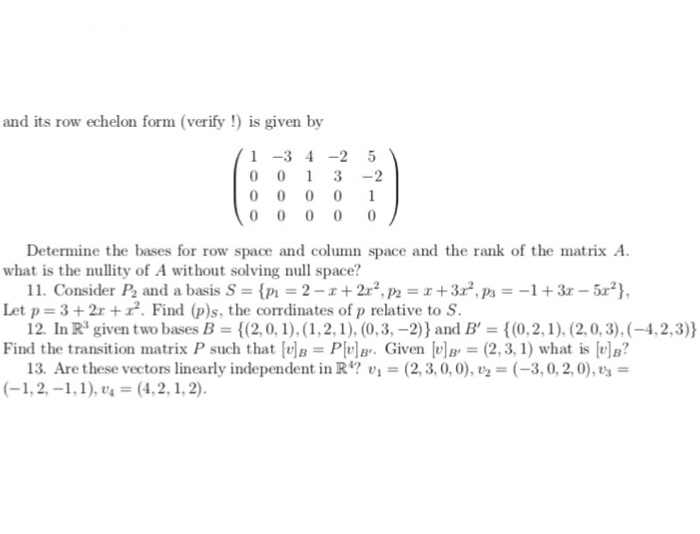and its row echelon form (verify ) is given by 1-3 4-2 5 0 01 3- what is the nullity of A without solving null space? Let p 3+2r+. Find (p)s, the corrdinates of p relative to S. Find the transition matrix P such that [tle = Plula.. Given lula, = (2,3, 1) what is lul? Determine the bases for row space and column space and the rank of the matrix A 11. Consider Pa and a basis S-in-2-r + 2r2, P2-х +312, p,--1 + 3-5r2), 12. In R3 given two bases B (2,0,1),,2), (0,3,-2) and B 0,2,1), (2,0,3),(-4,2,3)) 13. Are these vectors linearly independent in R1? (2,3,0,0), (-3,0,2,0),s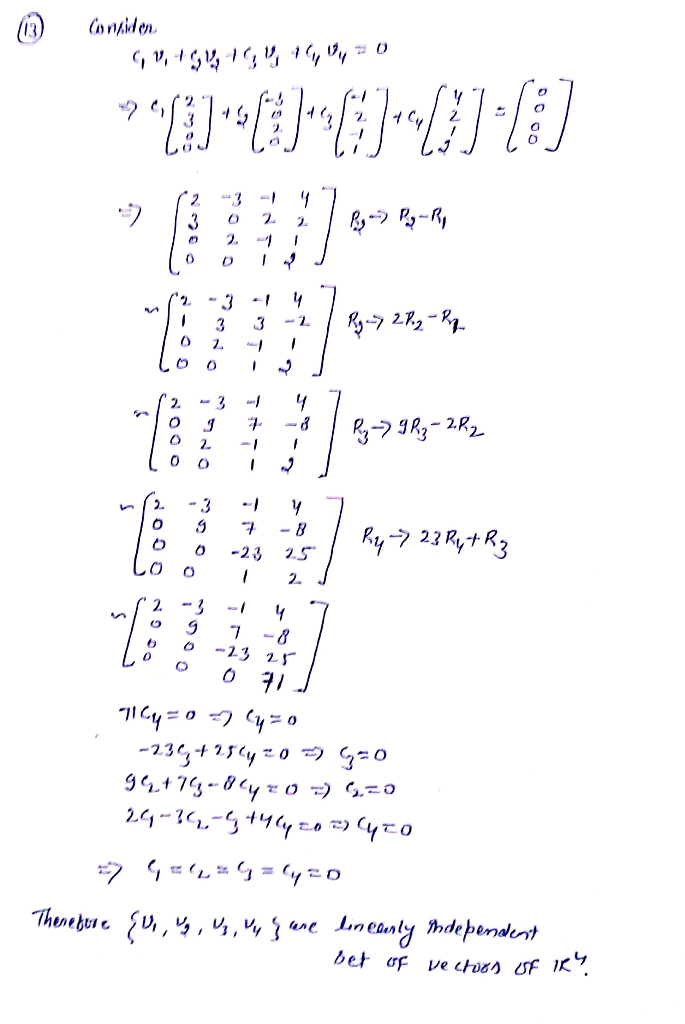#### Earn Coins

Coins can be redeemed for fabulous gifts.

Similar Homework Help Questions
• ### Given the matrix A and its reduced row echelon form R, answer the following questions. 4...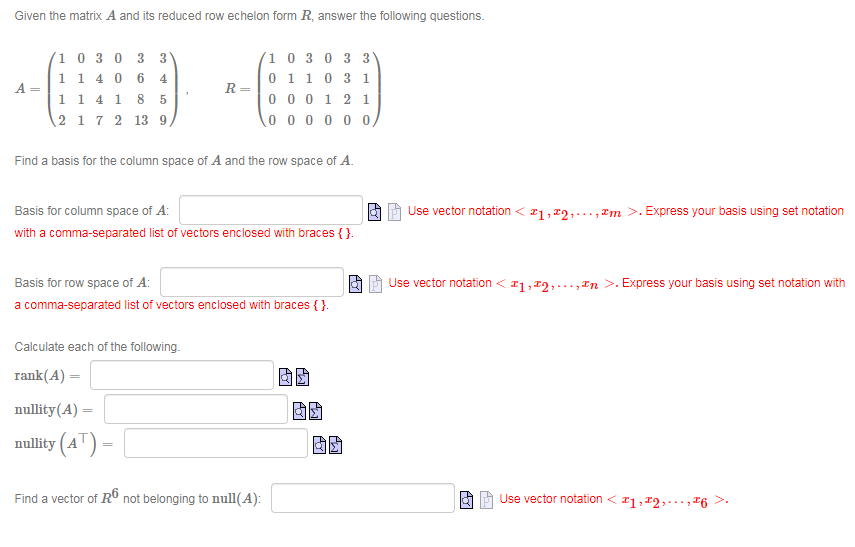Given the matrix A and its reduced row echelon form R, answer the following questions. 4 A 1 0 3 0 3 3 1 1406 1 1 4 1 8 2 1 7 2 13 9 R= 1 0 3 0 3 3 0110 31 000121 1000000 5 Find a basis for the column space of A and the row space of A. Ao Basis for column space of A: with a comma-separated list of vectors enclosed with braces {}....

• ### Given the matrix A and its reduced row echelon form R, answer the following questions. A=...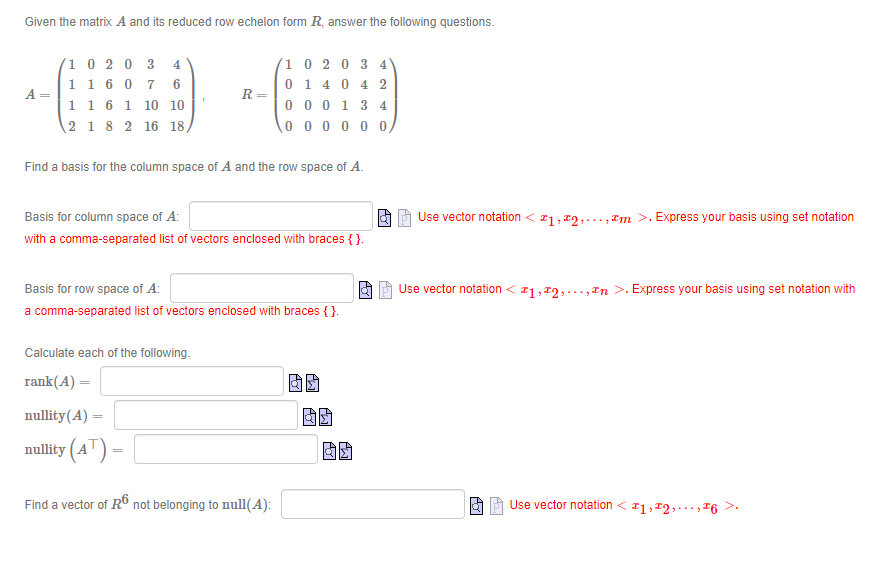Given the matrix A and its reduced row echelon form R, answer the following questions. A= 10 20 3 4 1 1 60 7 6 11 6 1 10 10 2 1 8 2 16 18 R= 1 0 2 0 3 4 0 14 0 4 2 000134 000000 Find a basis for the column space of A and the row space of A. Basis for column space of A: with a comma-separated list of vectors enclosed with braces...

• ### Given the matrix A and its reduced row echelon form R, answer the following questions. A=...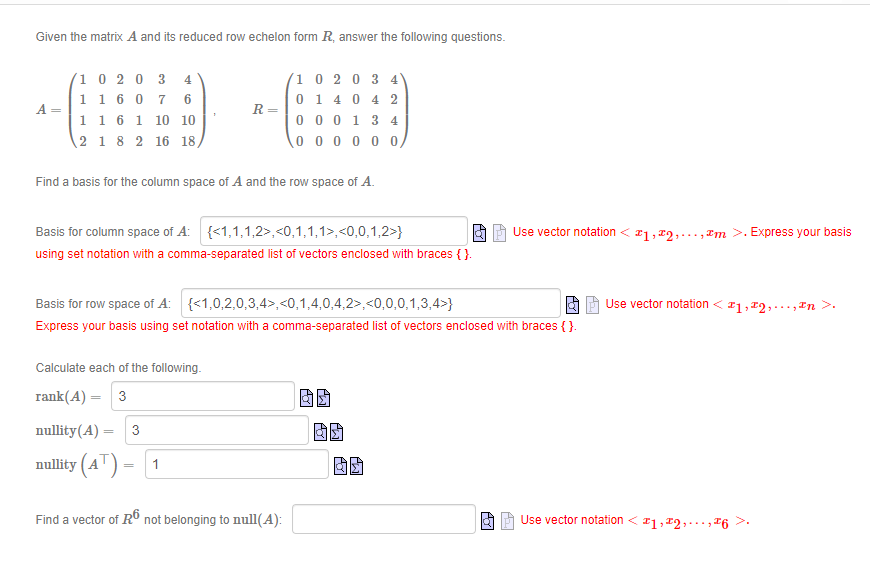Given the matrix A and its reduced row echelon form R, answer the following questions. A= 1 02 03 4 1 1 6 0 7 6 1 1 6 1 10 10 2 1 8 2 16 18 R= (10 20 3 4 01 4 0 4 2 000134 000000 Find a basis for the column space of A and the row space of A. Use vector notation <21,22,..., Im >. Express your basis Basis for column space of A:...

• ### Question 3 please answer clearly. A matrix A and its reduced row echelon form are given...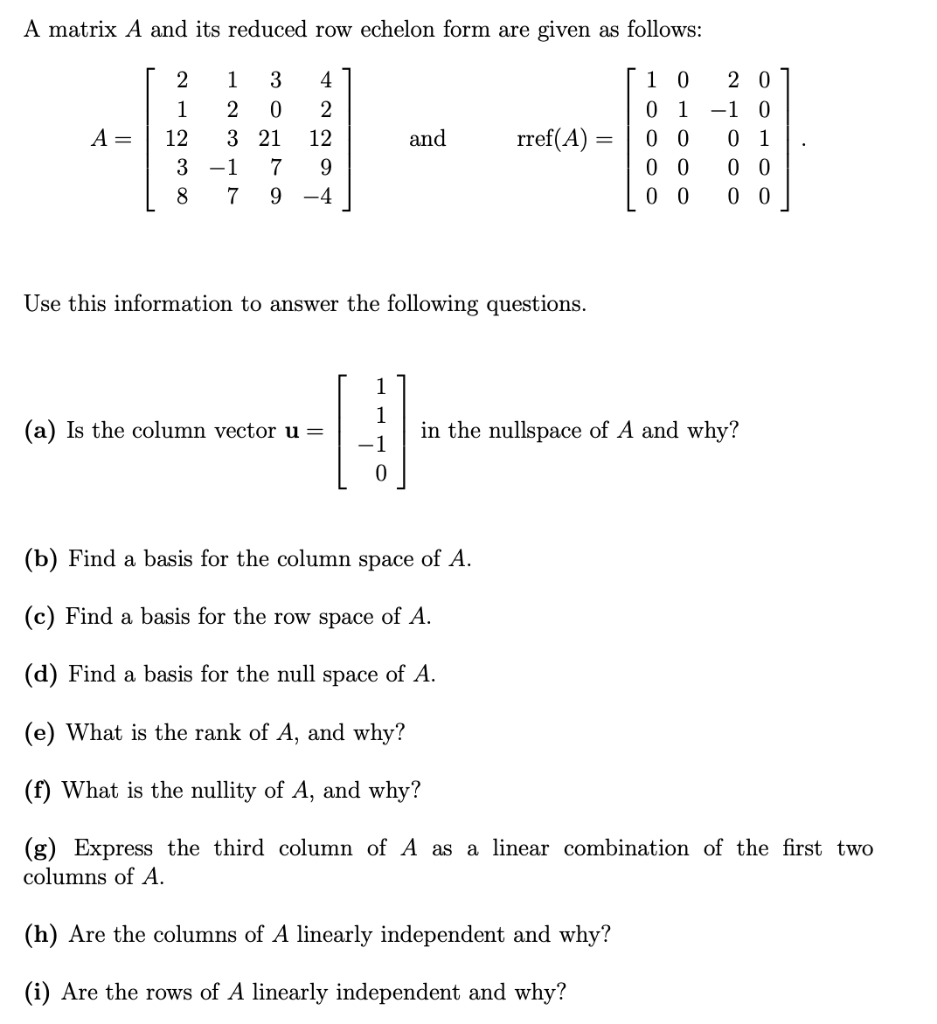Question 3 please answer clearly. A matrix A and its reduced row echelon form are given as follows: [ 2 1 3 41 | 1 2 0 2 A= 3 21 12 | 3 -1 7 9 18 7 9 -4 and rref(A) = [ 1 0 201 0 1 -1 0 0 0 0 1 0 0 0 0 | 0 0 0 0 Use this information to answer the following questions. (a) Is the column vector u= in...

• ### 1 The row-echelon reduced form of the matrix -22 3 1 3 2 0 2 0...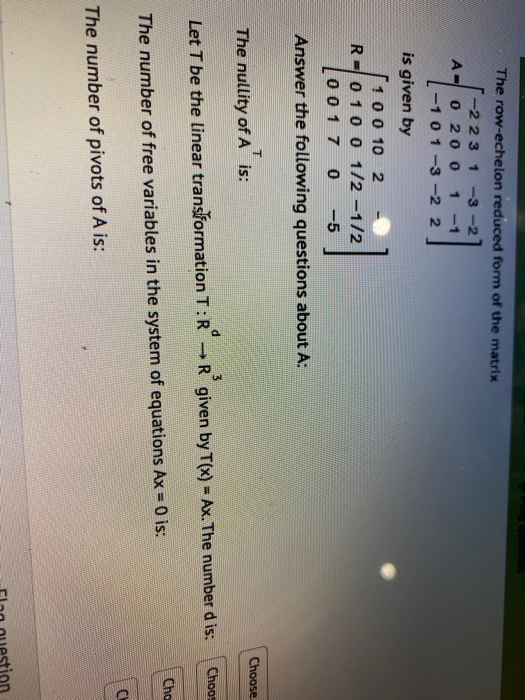1 The row-echelon reduced form of the matrix -22 3 1 3 2 0 2 0 0 -1 -1 0 1 -3 -2 2 is given by 10 0 10 2 R 0 10 0 1/2 -1/2 0 0 1 7 0 -5 Answer the following questions about A: Choose The nullity of A is: 3 Choo Let T be the linear transformation T:R → R given by T(x) = Ax. The number d is: Cha The number of free...

• ### 1. Consider the following matrix and its reduced row echelon form [1 0 3 3 5...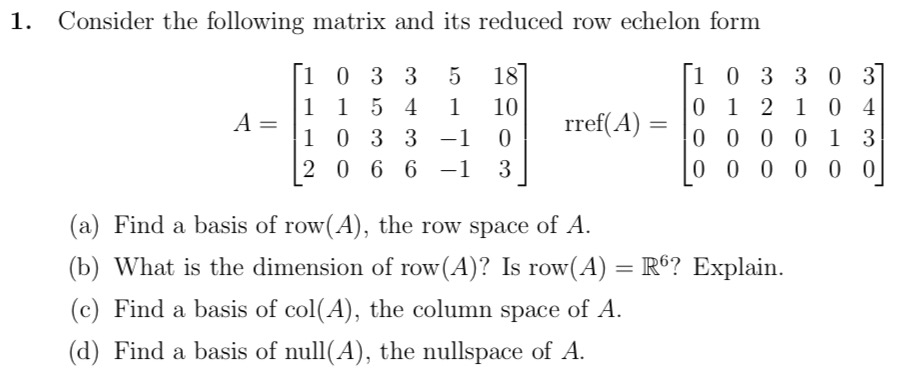1. Consider the following matrix and its reduced row echelon form [1 0 3 3 5 187 [1 0 3 3 0 37 1 1 5 4 1 10 0 1 2 1 0 - A=1 4 1 0 3 3 -1 0 rref(A) = 10 0 0 0 1 3 2 0 6 6 -1 3 | 0 0 0 0 0 0 (a) Find a basis of row(A), the row space of A. (b) What is the dimension...

• ### 4 1 1. The matrix A and it reduced echelon form B are given below. 1-2...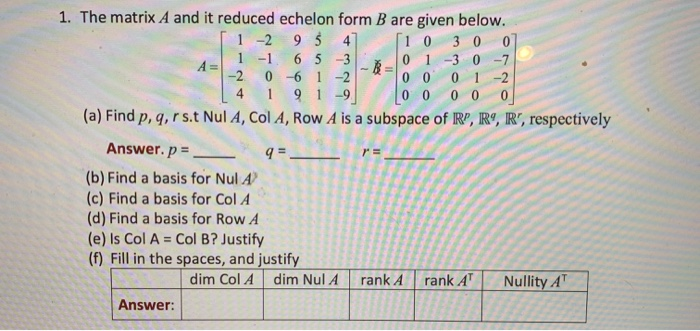4 1 1. The matrix A and it reduced echelon form B are given below. 1-2 95 4 10 3 0 0 1 -1 6 5 3 0 1 -3 0 -7 -2 0 -6 1 -2 0 0 0 1 -2 9 1-9 0 0 0 0 0 (a) Find p, q, r s t Nul A, Col A, Row A is a subspace of RP, R9, R', respectively Answer. p = 9= (b) Find a basis for Nul...

• ### 4 1 1. The matrix A and it reduced echelon form B are given below. 1-2...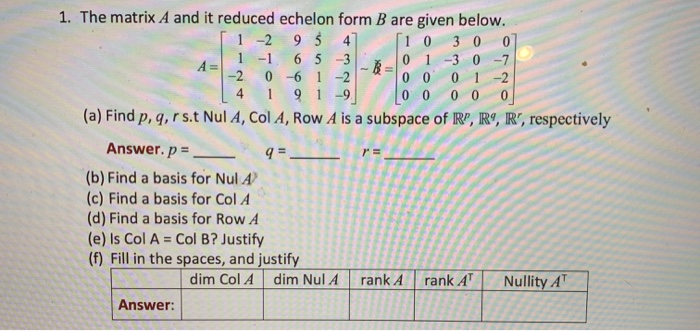4 1 1. The matrix A and it reduced echelon form B are given below. 1-2 95 4 10 3 0 0 1 -1 6 5 3 0 1 -3 0 -7 -2 0 -6 1 -2 0 0 0 1 -2 91-9 0 0 0 0 0 (a) Find p, q, rs. Nul A, Col A, Row A is a subspace of R", R9, R', respectively Answer. p = 9=- (b) Find a basis for Nul A (c) Find...

• ### 1. The matrix A and it reduced echelon form B are given below. 1-2 95 4...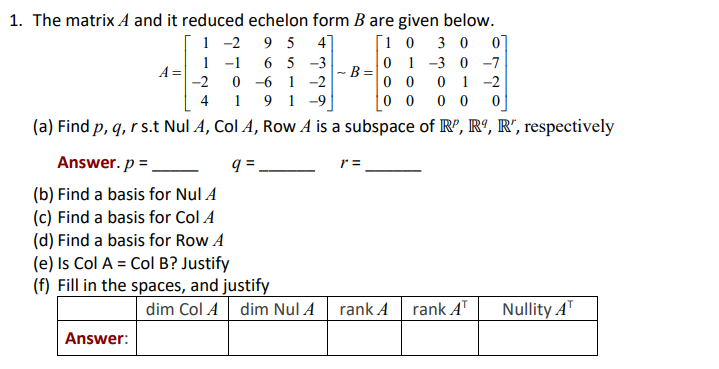1. The matrix A and it reduced echelon form B are given below. 1-2 95 4 [1 0 3 0 0 1 -1 6 5 -3 A= 0 1 -3 0 -7 -B= -2 0 -6 1 -2 0 0 0 1 -2 4 9 -9 0 0 0 0 0 (a) Find p, q, rs. Nul A, Col A, Row A is a subspace of R”, R9, R", respectively Answer.p = 9. r = (b) Find a basis for...

• ### Use row-reduction to put the following matrix to reduced row echelon form. 1 5 4 2...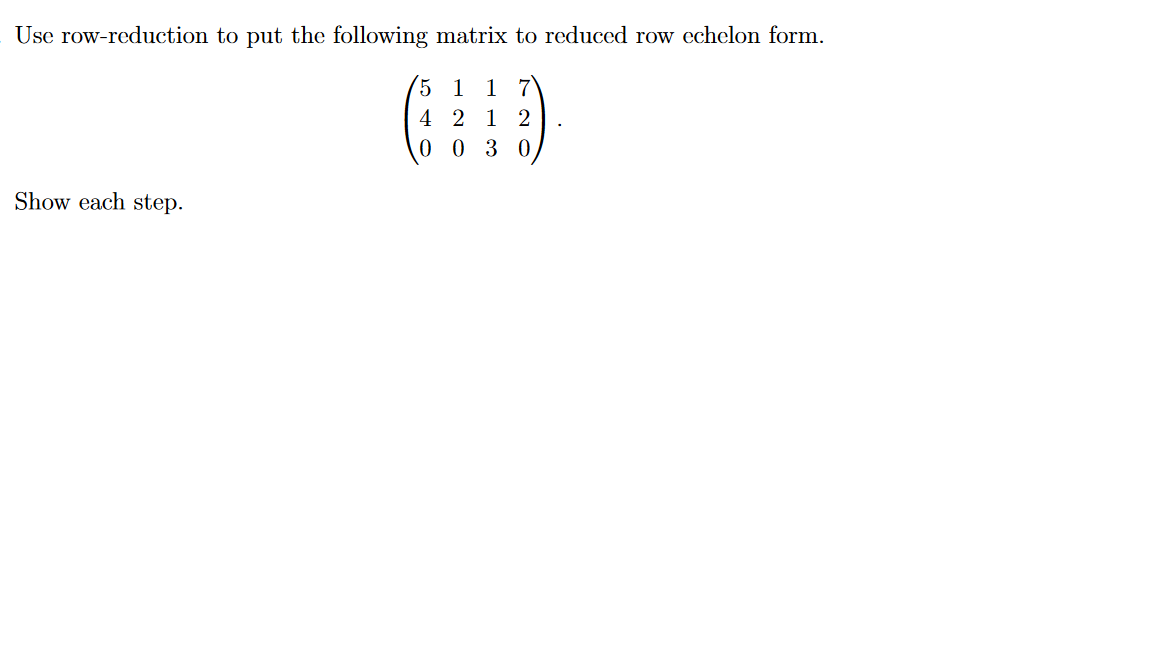Use row-reduction to put the following matrix to reduced row echelon form. 1 5 4 2 1 2 0 0 3 0 Show each step.